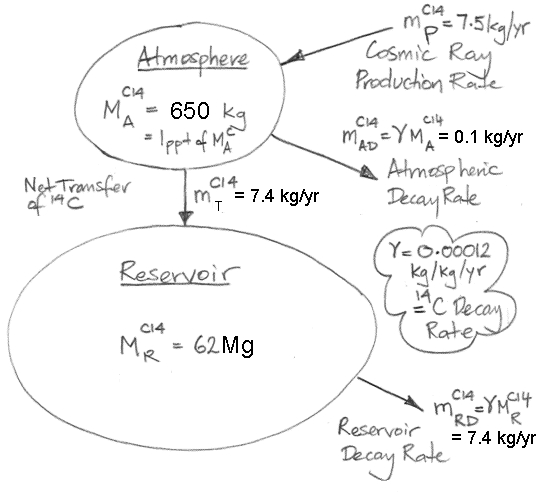## Monday, September 28, 2015

### Carbon-14: Removal from the Atmosphere

We are going to estimate the mass of carbon-14 in the Earth's atmosphere prior to our burning significant quantities of fossil fuels, and prior to our detonating atomic bombs. We will use the Earth's atmosphere at the end of the nineteenth century as an approximation to the atmosphere in its natural, equilibrium state, undisturbed by human activity.

The concentration of carbon dioxide in our equilibrium atmosphere is 300 ppmv (parts per million by volume, as estimated for the turn of the nineteenth century here). The total mass of the atmosphere is 5.3×1018 kg. (Atmospheric pressure is generated by the weight of the atmosphere per square meter, so divide sea-level atmospheric pressure by gravitational acceleration and multiply by the surface area of the Earth to obtain atmospheric mass.) In accordance with the gas law, the density of CO2 is 1.5 times higher than the density of air (the molar mass of CO2 is 44 g, and of air is 29 g). Thus 300 ppmv of CO2 in the atmosphere is the same as 450 ppm (parts per million by mass). The mass of CO2 in our equilibrium atmosphere is 2.4×1015 kg (450 ppm of 5.3×1018 kg). The molar mass of CO2 is 44 g, and that of carbon is 12 g, so the mass of carbon in the atmosphere is 6.5×1014 kg (2.4×1015 kg × 12 g ÷ 44 g). We will use petagrams (Pg) to represent large masses, where 1 Pg = 1012 kg = 1015 g. Our equilibrium atmosphere contains 650 Pg of carbon.

The concentration of carbon-14 in our equilibrium atmosphere is 1.0 ppt (parts per trillion by mass). Almost all carbon in the atmosphere is contained in CO2, so the mass of carbon-14 in our equilibrium atmosphere is 650 kg (650 Pg of CO2 × 1ppt). As we already showed, the equilibrium reservoir of carbon-14 on Earth is 62 Mg (7.5 kg/yr production by cosmic rays ÷ 0.00012 kg/kg/yr decay rate = 62,500 kg = 62 Mg). Of this reservoir, only 1% is to be found in the atmosphere. From now on, when we refer to the Earth's carbon-14 reservoir we will be referring to the 62 Mg that is not in the atmosphere.

The 650 kg of carbon-14 in our equilibrium atmosphere decays at 0.078 kg/yr (650 kg × 0.00012 kg/kg/yr) and is added to by cosmic ray production of 7.5 kg/yr. In order for the carbon-14 content of the atmosphere to remain constant, carbon-14 must pass out of the atmosphere at 7.4 kg/yr. Let us suppose, for the sake of argument, that this 7.4 kg/yr does not pass into the carbon-14 reservoir. In that case, the 7.4 kg/yr goes somewhere else, and a new reservoir starts to build up, while the existing reservoir decays, which would mean that our carbon-14 reservoir would not be in equilibrium, which contradicts our observation that the reservoir had millions of years to reach equilibrium before the nineteenth century. Thus 7.4 kg/yr of carbon-14 must pass directly from the atmosphere into the reservoir. It could be that the reservoir contains many sub-divisions communicating with one another in complex ways, but this does not alter the fact that 7.4 kg of carbon-14 is passing out of the atmosphere and into the reservoir every year.

The figure below illustrates the origin and fate of Carbon-14 on Earth. We use M for mass, and m for mass flow. We use subscript A for atmosphere, R for reservoir, D for decay, T for transfer, and P for production. Superscript C14 means carbon-14.Where is the Earth's carbon-14 reservoir? How does it acquire 7.4 kg of carbon-14 from the atmosphere every year?